# Toeplitz Matrix

## Toeplitz Matrix in C Language with Examples:

In this article, I am going to discuss Toeplitz Matrix in C Language with Examples. Please read our previous article, where we discussed TriDiagonal and TriBand Matrix in C Language with Examples.

##### Toeplitz Matrix:

This is a Toeplitz Matrix of order 5×5.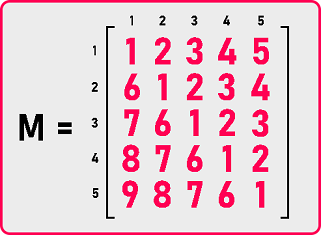This is having all non-zero elements then what is the property of this matrix? If we observe the elements in a diagonal the elements are the same.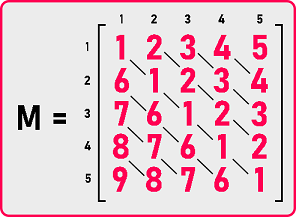Elements in a diagonal are same. So, when the elements are repeating, they are duplicating and there is some pattern followed which is: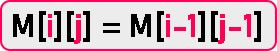We don’t have to store all non-zero elements. Then how many elements are sufficient to store in a single dimension array? 1st row and 1st column are sufficient to store an array: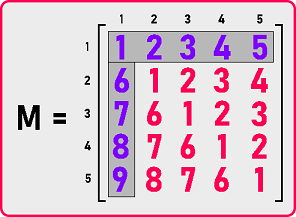The total number of Elements to be stored in the array are: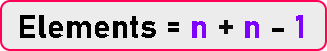So let us see how to represent this one. So, let’s take an array and store all these elements.

What should be the size of the array? The size will be n + n – 1

= 2n – 1

In this case, n is 5 so

= 2 * 5 – 1

= 9

So, we would take an array of size 9: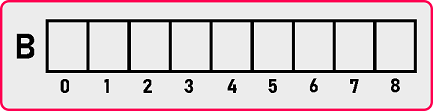Now how to represent these elements. We don’t have to store all the elements. First, we will store row and then columns.

###### 1st Row Elements: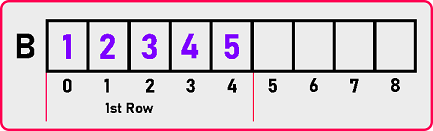###### 1st Column Elements: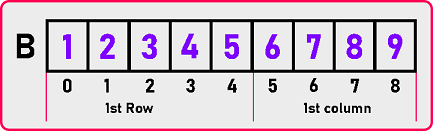We have done the mapping of the elements. Now, what should be the formula for retrieving the elements? See if the elements are in the upper triangular part, they are present in a row. And the elements are in lower triangular elements than they are in a column. So how do we identify the elements here? For retrieving the elements, the conditions are:

Index (M [i][j]):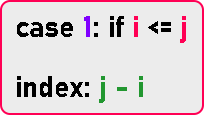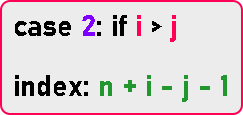So, these are the required formulas. Now let’s see the code part:

##### Toeplitz Matrix Code in C Language:
```#include <stdio.h>
#include <stdlib.h>

struct Matrix
{
int *B;
int n;
};

void Set (struct Matrix *m, int i, int j, int y)
{
if (i <= j)
{
m->B[j - i] = y;
}
if (i > j)
{
m->B[m->n + i - j - 1] = y;
}
}

int Get (struct Matrix m, int i, int j)
{
if (i <= j)
{
return m.B[j - i];
}
if (i > j)
{
return m.B[m.n + i - j - 1];
}
return 0;
}

void Display (struct Matrix m)
{
int i, j;

printf ("\nMatrix is: \n");

for (i = 1; i <= m.n; i++)
{
for (j = 1; j <= m.n; j++)
{
if (i <= j)
{
printf ("%d ", m.B[j - i]);
}
else if (i > j)
{
printf ("%d ", m.B[m.n + i - j - 1]);
}
else
{
printf ("0 ");
}
}
printf ("\n");
}
}

int main ()
{
struct Matrix M;
int i, j, y;

printf ("Enter Dimension of Matrix: ");
scanf ("%d", &M.n);
M.B = (int *) malloc ((2 * M.n - 1) * sizeof (int));

printf ("Enter all the elements of the matrix:\n");
for (i = 1; i <= M.n; i++)
{
for (j = 1; j <= M.n; j++)
{
scanf ("%d", &y);
Set (&M, i, j, y);
}
}

Display (M);
}
```
###### Output: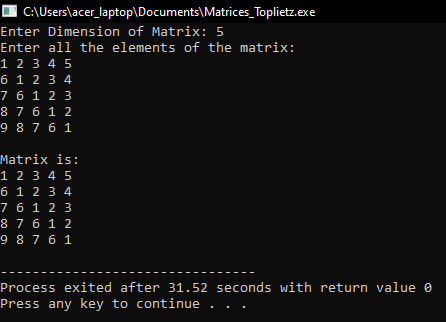In the next article, I am going to discuss Menu Driven Program for Matrices with Examples. Here, in this article, I try to explain Toeplitz Matrix in C Language with Examples and I hope you enjoy this Toeplitz Matrix in C Language with Examples article.